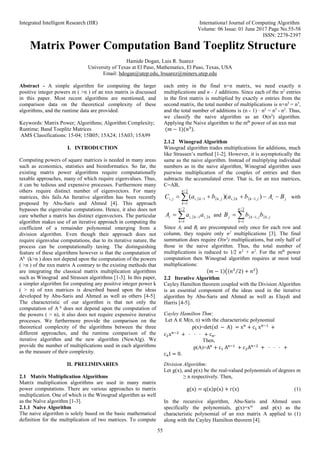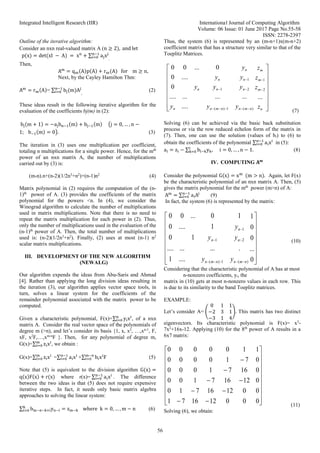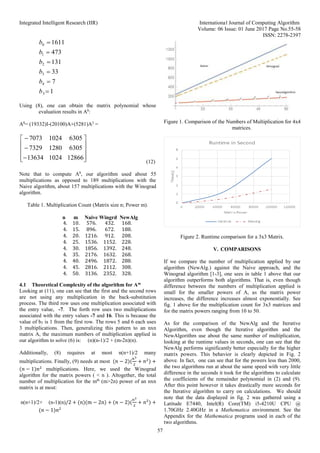Diese Präsentation wurde erfolgreich gemeldet.

# Matrix Power ComputationBand Toeplitz Structure

Anzeige
Anzeige
Anzeige
Anzeige
Anzeige
Anzeige
Anzeige
Anzeige
Anzeige
Anzeige
Anzeige×

1 von 4 Anzeige

# Matrix Power ComputationBand Toeplitz Structure

Abstract - A simple algorithm for computing the larger positive integer powers m (>n) of an nxn matrix is discussed in this paper. Most recent algorithms are mentioned, and comparison data on the theoreticalcomplexity of these algorithms, and the runtime data areprovided.

Abstract - A simple algorithm for computing the larger positive integer powers m (>n) of an nxn matrix is discussed in this paper. Most recent algorithms are mentioned, and comparison data on the theoreticalcomplexity of these algorithms, and the runtime data areprovided.

Anzeige
Anzeige